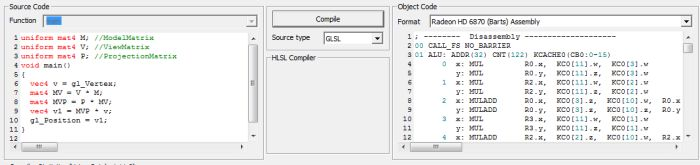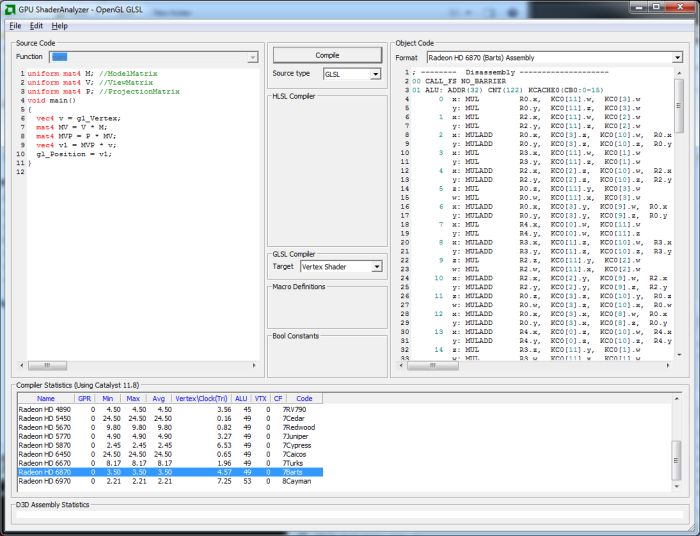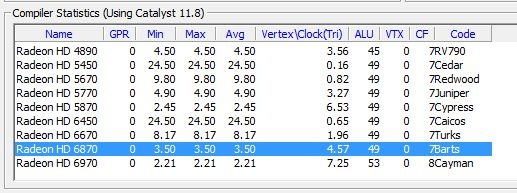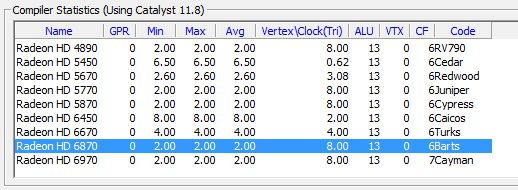# How to Compute the Position in a GLSL Vertex Shader (Part 2)(*** UPDATED ***)Two days ago, I published a simple code snippet about how to compute the position and normal in the vertex shader in OpenGL (GLSL) and Direct3D (HLSL). The aim was to show how to use the different model, view and projection matrices to compute gl_Position, especially the order of matrices multiplication in OpenGL and Direct3D. Here is the GLSL vertex shader (named VS_A):

```uniform mat4 M; //ModelMatrix
uniform mat4 V; //ViewMatrix
uniform mat4 P; //ProjectionMatrix
void main()
{
vec4 v = gl_Vertex;
mat4 MV = V * M;
mat4 MVP = P * MV;
vec4 v1 = MVP * v;
gl_Position = v1;
}
```

This method constructs the final transformation matrix (MVP or ModelViewProjection) and multiply it by the vertex position in mesh local space (gl_Vertex). This method works fine but generates a lot of GPU instructions.Once compiled, the vertex shader VS_A generates 49 ALU instructions.

Daniel Rakos came with a faster solution:

```uniform mat4 M; //ModelMatrix
uniform mat4 V; //ViewMatrix
uniform mat4 P; //ProjectionMatrix
void main()
{
vec4 v = gl_Vertex;
vec4 v1 = M * v;
vec4 v2 = V * v1;
vec4 v3 = P * v2;
gl_Position = v3;
}
```

Once compiled in GPU ShaderAnalyzer, VS_B generates 13 ALU instructions:I quickly did a test in GeeXLab with a mesh (sphere) made up of 2 million faces and 1 million vertices:
– VS_A: 425 FPS
– VS_B: 425 FPS

I also did a test with a simple OpenGL demo (a simple win32 test app) with geometry instancing (10000 instances, 900 vertices per instances), with no difference between both vertex shaders. I expected a small difference. One explanation might be the simplicity of the scene. But I’m sure we’ll see the difference in more complex shaders / 3D scenes because there must be a gain in performance between 49 and 13 ALU instructions…

Update (2011.10.31):
I think I found one possible answer: the overhead due to the shader invocation and / or vertex fetch. Indeed, if the real workload of the vertex shader is too small, most of the time is wasted in other tasks such as vertex fectching or shader invocation, actually all tasks that come before and after the vertex shader execution. Then the difference between 13 and 49 ALU instructions is not visible.

To validate this idea, I increased the workload of the vertex shader and compared both following vertex shaders with a mesh made up of 1 million vertices ():

```uniform mat4 M; //ModelMatrix
uniform mat4 V; //ViewMatrix
uniform mat4 P; //ProjectionMatrix
void main()
{
vec4 v = gl_Vertex;
vec4 pos = vec4(0.0);
for (int i=0; i<100; i++)
{
mat4 MV = V * M;
mat4 MVP = P * MV;
vec4 v1 = MVP * v;
pos += v1;
}
gl_Position = pos / 100.0;
}
```

and

```uniform mat4 M; //ModelMatrix
uniform mat4 V; //ViewMatrix
uniform mat4 P; //ProjectionMatrix
void main()
{
vec4 v = gl_Vertex;
vec4 pos = vec4(0.0);
for (int i=0; i<100; i++)
{
vec4 v1 = M * v;
vec4 v2 = V * v1;
vec4 v3 = P * v2;
pos += v3;
}
gl_Position = pos / 100.0;
}
```

With the vertex shader A, the demo ran at 19 FPS. With the vertex shader B, the demo ran at 64 FPS.

Ouf, got it! The intuition was good: there is now a nice difference of speed between both vertex shaders.

## 6 thoughts on “How to Compute the Position in a GLSL Vertex Shader (Part 2)(*** UPDATED ***)”

1.dfdg

Instancing is specifically made to reuse calculations. Thus with using instancing you minimize the amount of alu that need to be done.

The benefit is going to be with a lot of shaders that need to be used at the same time or with large shader pipelines. Or do an ai demo where a bunch of actors intelligence is being done with using shaders.

2.sqrt[-1]

Maybe I missed something – but why is the model-view-projection matrix not calculated on the CPU and sent to the GPU as a single matrix?

99% of shader code I have seen does this.

3.JeGX Post Author

No, you missed nothing 😉 It’s just a code snippet to show the manipulation of matrices in the vertex shader. Sure you can compute the final MVP matrix in the host app (or use gl_ModelViewProjectionMatrix with non core profile) but in some cases, it can be useful to play with matrices directly in the shader.

4.ca\$per

Perhaps at some computation intense sitations the difference might be visible. For example skinning with a large number of bones or vertices.

5.JeGX Post Author

@ca\$per: yep, with a heavier workload, the difference is visible as I show it in the update.

6.marz

Don’t take me wrong, but this should be obvious to everybody, who knows a little about matrix-matrix and matrix-vector multiplication 🙂

It’s much less computations, if you multiply three times matrix and vector (completely something about 48 floating point multiply operations + some additions) than twice matrix and matrix + once matrix and vector (completely about 144 floating point multiply operation + some additions :)).